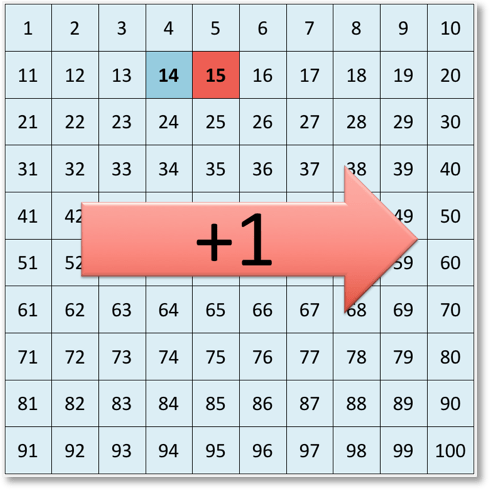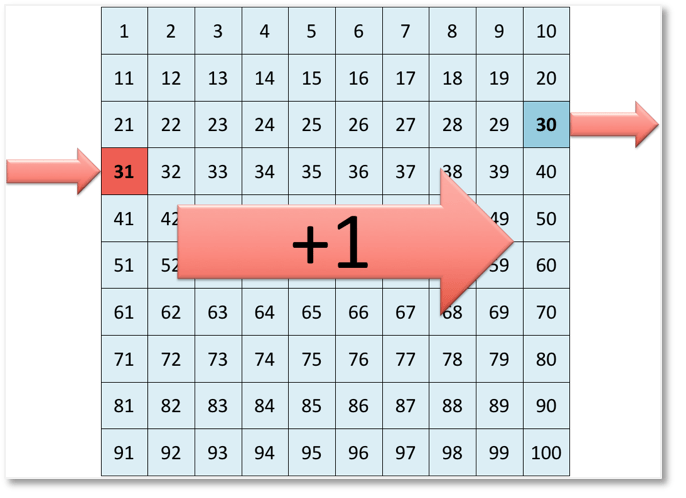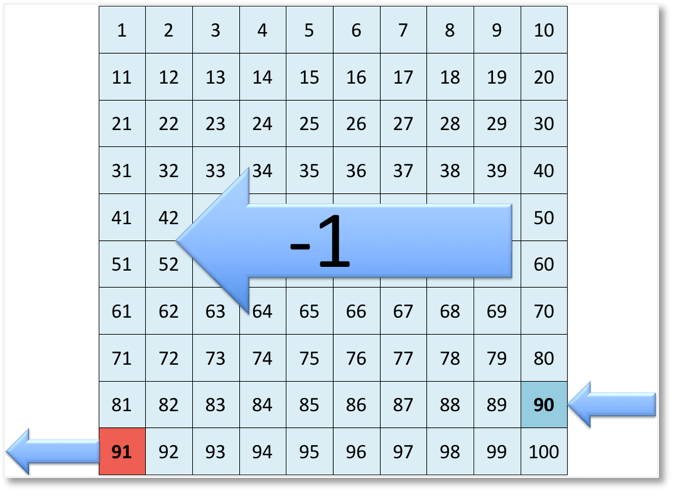# Number Grid: Adding and Subtracting One

Number Grid: Video Lesson – Maths with Mum

## How do we Add or Subtract using the Number Grid?

If we choose a number on the number grid and move one place to the right, we are adding 1.

For example:If we start at 14 and move one place to the right, we have added 1.

14 + 1 = 15.

If we begin with a number that is at the end of a row, we move to the beginning of the next row.

For example:If we begin with 30, to add 1, we move to the beginning of the next row.

30 + 1 = 31.

If we choose a number on the number grid and move one place to the left, we are subtracting 1.

For example:If we start at 77 and move one place to the left, we have subtracted 1.

77 – 1 = 76.

If we start with a number that is at the beginning of a row and subtract one, we move to the end of the previous row.

For example:If we begin with 91, to subtract 1, we move to the end of the previous row.

91 – 1 = 90.